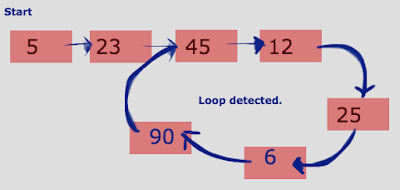Showing posts with label How to detect loop in a linked list or not. Show all posts
Showing posts with label How to detect loop in a linked list or not. Show all posts## How to detect loop in a linked list or not

We have seen lot of posts related to LinkedList like,

Finding N'th node from the end of a Linked List
Find the middle node of a given linked list

now lets see how to detect or find whether the linked having loop or not. For example if we traverse the linked list then it will be indefinite and falls into a cyclic loop of entire linked list or within the linked list.
Lets create simple linked list by having loop and by another method lets try to find the loop present or not. We can solve/ find the loop in linked list using multiple ways like

• Storing the NODE in HashSet and checking for each put whether we have same NODE already in HashSet or not. If its present then loop present.
• Next keep 2 pointers to traverse the nodes like 1st pointer will traverse 1 NODE at a time and 2nd pointer will traverse 2 NODE's at time. At some point 1st and 2nd pointer's will be same and then loop present or else loop not present.
Lets see both implementation in a single program with 2 differnt methods.

Example's:

array[] = { 11, 12, 13, 14, 15, 16, 17, 18 }
loopValue = 14
Here LinkedList created with loop from 14 to 18

Output: Loop present

array[] = { 1,2,3,4,5,6,7,8,9}
loopValue = 1
Here LinkedList created with loop from 1 to 9

Output: Loop present

array[] = { 10,20,30,40,50,60,70,90,100 }
loopValue = 25
Here LinkedList created and we don't have any node with value 25.

Output: Loop NOT present

```import java.util.HashSet;

class NODE {

int data;
NODE next = null;

public NODE(int data) {
this.data = data;
}
}

public static void main(String[] args) {

int array[] = new int[] { 11, 12, 13, 14, 15, 16, 17, 18 };
int loopValue = 14;

//int array[] = new int[] { 1, 2, 3, 4, 5, 6, 7, 8, 9 };
//int loopValue = 1;

//int array[] = new int[] { 10, 20, 30, 40, 50, 60, 70, 90, 100 };
//int loopValue = 25;

// Create linkedlist based all array values

String output1 = obj.findLoopUsingHashSet(start);
String output2 = obj.findLoopUsing2Pointers(start);

System.out.println("Using HashSet    : "+output1);
System.out.println("Using 2 pointers : "+output2);

}

/*
* Create LinkedList and return the start pointer/node
*/
public NODE createLinkedList(int[] array, int loopValue) {

NODE start = null;
NODE tmp = null;

// Pointer to hold the loop start node

for (int i : array) {

tmp = new NODE(i);

if (start == null) {
start = tmp;
} else {
NODE mover = start;
while (mover.next != null) {
mover = mover.next;
}
mover.next = tmp;
}

// pointer of the node where loop starts
if (i == loopValue) {
}
}

// Create loop from last node to linknode which has loopValue.
}

return start;
}

public String findLoopUsingHashSet(NODE start) {

HashSet<NODE> hs = new HashSet<NODE>();

while (start != null) {
if (hs.contains(start))
return "Loop present";

start = start.next;
}
return "Loop NOT present";
}

public String findLoopUsing2Pointers(NODE start) {

NODE first = start, second = start;
while(second != null && second.next != null && second.next.next != null) {
first = first.next;
second = second.next.next;
if(first == second)
return "Loop present";
}
return "Loop NOT present";
}
}
```

OUTPUT:

```Using HashSet    : Loop present
Using 2 pointers : Loop present
```